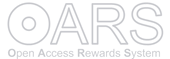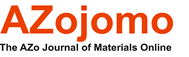DOI : 10.2240/azojomo0318

# Estimating Life of Reinforced Concrete Corroded by Chloride Ingress

## Laurie Aldridge and Frank Collins

This is an AZo Open Access Rewards System (AZo-OARS) article distributed under the terms of the AZo–OARS https://www.azom.com/oars.asp which permits unrestricted use provided the original work is properly cited but is limited to non-commercial distribution and reproduction.

Submitted: December 9th, 2011
Volume 8 January 2012

## Topics Covered

Abstract
Keywords
Introduction
Proposed Method of Service life Calculation
Service Life Estimation with No Estimated Surface Chloride Concentration
Method Sensitivity
Discussion
Conclusions
References
Contact Details

## Abstract

There is a worldwide need to estimate the service life of reinforced concrete exposed to chloride ions. That service life of reinforced concrete is limited by chloride induced corrosion of reinforcement steel is well known with the costs of such corrosion significant. Chloride ingress has long been modelled by equations based on Fick’s second law where the chloride profile into the concrete cover is related to; the time of diffusion, the distance of ingress, the diffusion constant of the chloride ion in that concrete (D), and the concentration of the chloride ion on the concrete surface (S).

In order to predict the service life of the concrete cover over reinforcement steel then dependence of both D and S with time has to be modelled. There is no simple method available to engineers to routinely predict service life by determination of the time dependence of D & S. This paper introduces such a method that determines D and S after chloride penetration at 28, 56, 112, & 224 days. The method produces the data needed for simple service life predictions with all calculations able to be carried out on an Excel spreadsheet. In this report the robustness of the method was tested using Monte Carlo methods where from errors in D & S the errors in estimating service life was computed.

## Keywords

Service Life, Durability, Reinforced Concrete, Chloride Ingress

## Introduction

There is a worldwide need to estimate the service life of reinforced concrete exposed to chloride ions. That service life of reinforced concrete is limited by chloride induced corrosion of reinforcement steel is well known with the costs of such corrosion significant. Much of Australia’s built infrastructure is concentrated around coastline, and vulnerable to the corrosive effect of exposure to chloride-laden sea water, groundwater, surface water and spray. If unchecked, corrosion deterioration can lead to loss of functionality, unplanned maintenance/remediation/replacement, and in the worst cases, loss of structural integrity and resultant safety risks. The community burden arising from restoration of deteriorated infrastructure is 2-4% GDP/annum [1-2].

Knowledge of the chloride ingress into concrete is needed before reliable estimates of service life can be calculated. Aldridge  reviewed the use of Fick’s second law by a number of workers, to model chloride profiles in concrete at different times of exposure to chloride ion. This law produced an equation for calculating at a specific times the chloride composition as a function of distance from the surface assuming both S and D were time independent as

C(x) = S (1 – erf(x/ {2 sqrt(t D)})           (1)

Where; C(x) is the chloride profile or the concentration of the chloride (given sometimes as the weight % of the concrete but should be expressed as weight % of the dry binder {cement or cement & supplementary cementitious materials}) at a distance x [mm], S is the concentration of the chloride at the surface of the penetrating chloride in the same units as C(x), t is the time in years of the exposure of the concrete to the chloride ion D is the diffusion coefficient of the chloride expressed in mm2/years.

This equation is not useful at it assumes that both S & D are independent of time which is not the case (3). Both S and D must be considered to be dependant on time as predicted by a power law where

D(t) = D0(t/t0)-n      (2)

S(t) = S0(t/t0)m       (3)

The values n and m are powers lying between 0 and 1. Note the difference in signs in equations 2 & 3 is due to the fact that S increased with time while D decreased with time.

Combining Equations 1, 2, & 3 gives the equation (4).

C(x) = S0(t/t0)m (1 – erf(x/{2 sqrt(t D0(t/t0)-n )})      (4)

As was pointed out in the review , this equation is the basis of a number of models of service life prediction. It is unfortunate that none of the models reviewed gave a reliable method of estimating the n or m. Thus unreliable time dependant estimates of both D and S are found suggesting that only flawed values of service life predictions can be produced without accurately determining D0, S0, n and m for the actual concrete relevant for this service life.

In this work we have suggested a method for determining D0, S0, n and m for a concrete. In order to estimate the precision of the service life a calculated data set of chloride values has been generated. The methods and data used are in the excel spreadsheet that is available from the authors.

To highlight the boundary conditions for computing the service life the chloride ingress has been calculated under a number of conditions. Using equation 1, at a time of 100 years, with S ranging from 2.78 to 6.95 wt% binder the concentration of chloride at the depth of 70mm was calculated as a function of D. These results plotted in Figure 1. When D=16 mm2/year & S=2.78 wt% binder the concentration of chloride ions at a cover of 70 mm is 0.6 wt% binder at 100 years. Thus for concrete with a cover of 70 mm with S at 2.73 and D 16 mm2/year the service life would be 100 years if it is assumed here that the critical concentration of chloride needed to initiate chloride corrosion is 0.60 chloride weight % of the dry binder. This assumption follows Bamforth  and the limitations inherent in this assumption are discussed in his publication and the limit is shown as a vertical line in figure 1.

Hence it is possible to compute service life (based on the time at which corrosion would be initiated) at time t for a cover depth xc when D0 S0 n and m are known if it is assumed that when the chloride concentration of the cover depth is greater than 0.6%. This calculated service life would be a conservative estimate as corrosion would be expected to only occur after this time. Note however that surface chloride is very dependant on exposure environment. For example, in a splash zone, the surface chloride (S) will be considerably greater due to wetting & drying compared concrete that is 100% air-exposed .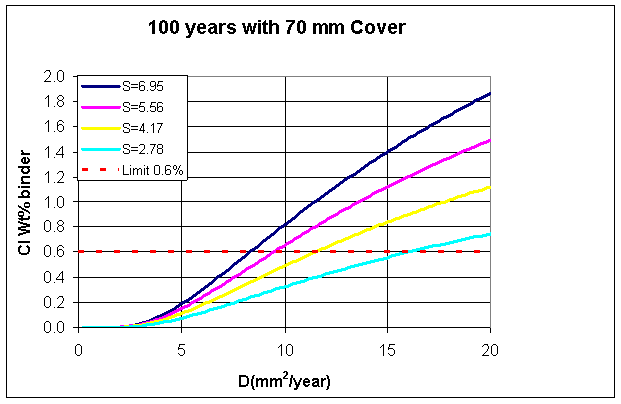Figure 1. Chloride concentrations at 70mm cover after 100 years as a function of the surface chloride(S) and chloride diffusion coefficient D.

## Proposed Method of Service life Calculation

Measurements of D and S in triplicate should be made at 28, 56, 112 and 224 days using a modification of Nordtest (1995) NT Build 443 “Concrete, Hardened: Accelerated Chloride Penetration”. This test is to be modified so that the curing time at the end of the measurement is the time of measurement. The natural log D values against natural log T from which n and D0 can be derived. Similarly S0 and m could be obtained from similar plots of S(t).

Below in tables 1 & 2 two examples are given to illustrate the procedure. The source of the data was generated from the fits given in  to penetration profiles given later in table (6) of this paper . From this data D and S were calculated and added to random numbers distributed in such a way to simulate errors expected from the determination of S and D. It can be assumed that the precision of the values are such that the % coefficient of variation of D is 10% while the % standard deviation of S is ~1% of the weight of concrete (which assuming that about a sixth of the weight of concrete is due to the binder would imply that the % coefficient of variation of S is about ~5% (%wt Binder). Note that the precision of determination of D and S (within and between laboratories) are discussed in references [6-7].

The times (28, 56, 112 and 224 days) were based on unpublished work by A. Jajou Kirk Vessalas, K. Fernado, and L.P. Aldridge. Although it is theoretically possible to omit the 224 days data (and that this can be done is illustrated in the approximate fit shown in the excel spreadsheet it is strongly recommended that the full four times be used. Indeed as will be shown later accurate values of D should be estimated at 10 years and for such extrapolations a data should be taken till at least 224 days.

Table 1. Example 1 - Three independent values of D(mm2/year) & S (Cl % Binder) determined at 28, 56, 112 & 224 days. Averaged estimates of the triplicate measurements are also shown. For the data source in Tables 1 & 2 see the text above.

Time Days Years D1(T) D2(T) D3(T) DAv(T) S1(T) S2(T) S3(T) SAv(T)
28 0.077 160 165 149 158.7 2.35 2.5 2.43 2.43
56 0.153 104 75.5 105 95.2 2.71 2.52 2.7 2.64
112 0.307 63.8 64.9 67.2 65.3 3.12 2.75 3.02 2.96
224 0.614 33.1 41.7 43.4 39.4 3.49 3.33 3.42 3.41

Table 2. Example 2 - Three independent values of D(mm2/year) & S (Cl % Binder) determined at 28, 56, 112 & 224 days. Averaged estimates of the triplicate measurements are also shown

Time Days Years D1(T) D2(T) D3(T) DAv(T) S1(T) S2(T) S3(T) SAv(T)
28 0.077 248 243 275 255.2 1.90 1.99 1.88 1.92
56 0.153 220 201 213 211.2 2.00 1.86 1.80 1.89
112 0.307 230 185 180 198.4 1.76 1.94 1.93 1.88
224 0.614 165 147 144 152.0 2.09 1.79 1.93 1.94

Plots from the data of examples 1 & 2 are found in Figures 2 & 3. An Excel spreadsheet to carry out regression of equations 5 and 6 and the values obtained for D0, S0, n & m are given in table 3 (for Example 1) and table 4 (for Example 2).

Ln(D/D28) = slopeD Ln(T/T0) + InterceptD      (5)

Ln(S/S28) = slopeS Ln(T/T0) + InterceptS      (6)

Here; D28 and S28 are the diffusion and surface concentration at T0 of 28 days, n=-slopeD, m=slopeS, D0= D28*exp(InterceptD), & S0= S28*exp(InterceptS). As noted all of the regressions were carried out on the plots of Ln(T/T0) v’s Ln(D/D28)

Table 3. Example 1 - Regression data of the averaged values with the derived values of n, m, D0 & S0

TimeDays Ln(T/T0) Ln(D/D28) Ln(S/S28)
28 0.000 0.0000 0.0000
56 0.693 -0.5112 0.0855
112 1.386 -0.8875 0.1998
224 2.079 -1.3924 0.3412
SlopeD -0.6569 SlopeS 0.1641
InterceptD -0.0147 InterceptS -0.0140
N 0.66 m 0.16
D0 156 S0 2.39

Note that from the data fitted in Example 1 S/S0 appears to vary with time which is not the case for Example 2 where S/S0 appeared to be a constant (and m was set to 0).

It should be noted that either natural logarithms (that is log to the base of e) or logarithms to the base 10 could be used. In actual fact in this paper natural logarithms to the base e were used. The slope of the plot of ln(T/T0) v’s ln(D/D0) gives –n while the D0 is computed from DAv(28days)*exp(InterceptD). Similarly m and S0 are computed from the plot of ln(T/T0) v’s ln(S/S0). The fitted valued are found in tables 3 & 4 and the plots are shown in figures 2 & 3.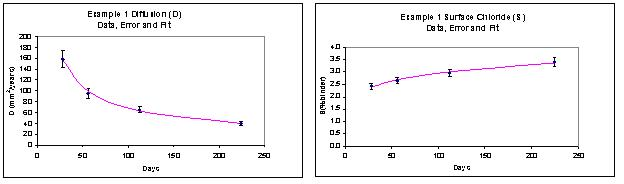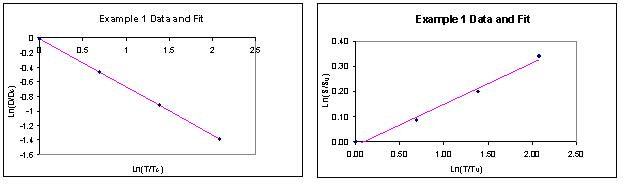Figure 2. Data and plots for the regression from Example 1

Table 4. Example 2 - Regression data of the averaged values with the derived values of n, m, D0 & S0

TimeDays Ln(T/T0) Ln(D/D28) Ln(S/S28)
28 0.000 0.0000 0.0000
56 0.693 -0.1890 -0.0192
112 1.386 -0.2517 -0.0246
224 2.079 -0.5183 0.0069
SlopeD -0.2333 SlopeS 0.0022
InterceptD 0.0029 InterceptS -0.0115
N 0.23 m 0.00
D0 159.13 S0 2.40

Using the values of D0, S0, n & m given in tables 3 and 4 it is possible by equation 4 to compute the chloride concentrations expected at cover of 70mm as a function of time. This estimate is plotted in Figure 4 in the figure shown in the left hand side. We plot the assumed limit of initiating the corrosion and as before suggest that service life of concrete with 70 mm cover can we estimated from when the % Cl crosses the time. In example 1 this will be greater than one hundred years and for example 2 it will be 52 years. It should be noted that in the case of example 1 the chloride concentration is barely visible on the graph.

We will suggest latter that equations 2 & 3 may only be valid till 10 years. If this is the case then the chloride values will be significantly lower and the difference is shown by the graph on the right hand side of Figure 4. In this case for example 2 the service life would have been 36 years.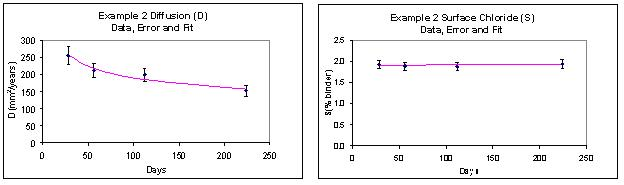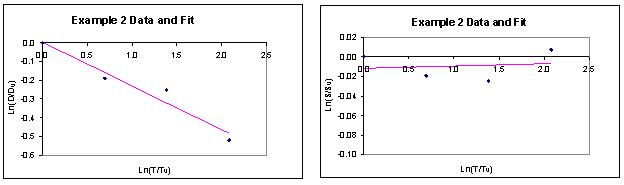Figure 3. Data and plots for the regression from Example 2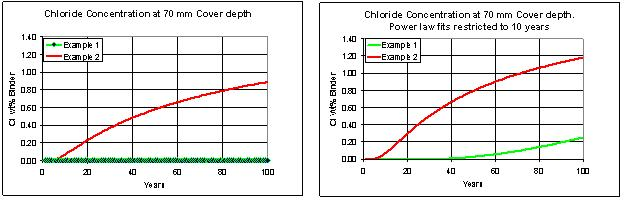Figure 4.Concentration of chloride as a function of time at cover depth 70 mm from equation 4 using the values derived from Examples 1 (in green) & Example 2 (in red). As discussed in the text there is some doubt about the validity of the power law fits (i.e D(t)=D0(t/t0)-n & S(t)=S0(t/t0)-n) shown on the left hand side of the graph. Hence the concentrations were recalculated assuming that the power law fit was only valid up to 10 years and D(t) and S(t) were computed using the approximation D(t)=D0(10/t0)-n & S(t)=S0(10/t0)-n. The concentrations of Cl which were significantly higher are shown in the graphs on the right hand side.

## Service Life Estimation with No Estimated Surface Chloride Concentration

There are significant advantages in using Nordtest (1999) NT Build 492 “Chloride Migration Coefficient from Non-Steady-State Migration” rather than the NT Build 443. It is faster, taking only 24 hours to carry out, it is more precise and hence it is ideal for quality control. However Build 492 only measures D and not S. Theoretically by using assumptions on the values of S it would be possible to calculate limiting values of the service life. For example it was been assumed by the authors of fib Bulletin 34  that S can be used as a constant value of 2.79. The importance of having the correct value of S was stressed in the Chlortest project  and the folly of using the 2.79 estimate to compute service life when the correct value is higher is illustrated in Figure 1. However it is possible that if conservative assumptions are made then it is possible to estimate lower bounds of service life. For example El Cherkawi  used a number of estimates of S in his estimates of service life and it would perhaps be possible to use NT Build 492 and a “worst case” for S (say 6.4) to estimate a service life.

Following El Cherkawi  and assuming that there is a cover depth of 70mm and the critical chloride concentration is 0.6% then a calculation of D and S needed to attain service lives of 50 and 100 years was carried out and the results are given in Table 5 at various values of S which are expressed as a ratio of the fib 34 estimate of S (2.74).

Table 5. Values of S and D needed to attain a 50 or 100 year service life

S Chloride Surface Concentration wt% binder Ratio of S to the fib 34  estimate of S D for 100 yr service life (mm2/year) D for 50 yr service life (mm2/year)
1.39 0.5 40 80
2.78 1 16 32
4.17 1.5 12 23
5.56 2 10 19
6.95 2.5 9 17
8.34 3 8 16

## Method Sensitivity

The proposed method of estimating service life relies on equations 2-4 to provide a semi-empirical fit of D and S with time so that realistic service lives can be evaluated for reinforced concrete. Such an approach relies on the time dependence of D and S. If values of D & S measured after only 90 days or even 1 years curing then the service lives of the reinforced concrete would be much shorter than expected. However concretes cured for such a period of time are generally deemed to be fully cured. In order to test the ability of the proposed model we have taken the values of D and S fitted by Aldridge  to the data of Lee Chisholm  for 4 concretes. This data and a summary of the concrete performance are given in table 6. The four concretes were made from New Zealand General Purpose (GP) OPC cement and Durcem Cement (DC) where 65% GGBFS acted as a cement replacement (The GGBFS or Ground Granulated Blast Furnace Slag was imported from Port Kembla NSW, Australia). Two different cement contents were used in the concretes with target loadings of 325 kg/m3 or 400 kg/m3 binder/concrete.

Table 6. Fitted Parameters and data from Lee and Chisholm . D0 (mm2/s) & S0 (wt % Cl with respect to binder) were fitted at 28 days. The water binder (w/b) Density (ρ) (kg/m3) & Compressive strength (MPa) at 28 days fc values were all taken from Lee and Chisholm .

D0 N S0 m w/b ρ fc
GP325 266 0.28 1.92 0.00 0.49 2403 54
GP400 159 0.28 1.77 0.06 0.40 2421 60
DC325 165 0.58 4.63 0.00 0.49 2442 39
DC400 15 0.61 2.37 0.18 0.44 2442 42

A comparison of the service lives calculated from the fits is given in table 7 (Row case 1a). However it is recognised that equations 2 & 3 cannot be extrapolated for 100 years. For some concretes power law fits of S would imply that at 100 years the concentration of surface chloride S would be greater than 100%. It can be suggested therefore that some limitations to the equations 2 and 3 are necessary. In the calculations give in table 7 two cases are given where D(T) and S(T) are computed at either 5 or 10 years. In an earlier paper it was shown that power law fits on data up to 10 years were acceptable and hence we suggest that this ten year limit be taken as reasonable. There is in our opinion no data suggesting that the power law fits beyond 10 years has any physical justification.

Somerville  has suggested that the basis of a performance plan would be to differentiate the expected service life into periods (in years) represented by; less than 5, 5-10,10-20, 20-40, 40-100, and greater than 100 years. Any model of service life should be able to determine which of these categories the concrete should fit. Methods for such forecasting rely on accurate estimates of the fitted parameters to obtain robust reliable estimates of future values. To test it this method would give acceptable results we have used a Monte Carlo method for simulating 100 sets of experimental data of D and S (at 28, 56, 112, & 224 days) with the errors defined in the previous section. These results were then fitted according to the defined method and the standard deviations of the simulated results calculated. The results are shown in table 7 and suggest that the suggested method would provide service life predictions which are precise enough to be used to differentiate concretes into the categories; less than 5, 5-10, 10-20, 20-40, 40-100, and greater than 100 years.

Table 7. Service lives with errors (standard deviations given in brackets) computed for the concretes of Lee and Chisholm  using different models. Case 1a assumed that the fits given in Table 6 were correct. Case 1b and 1c assumed that D(t) and S(t), computed assuming equations 2 & 3 where t = 5 or 10 years. Case 2 data were all calculated assuming that D(t) and S(t) were calculated at: (Case 2a) the time of service life, (Case 2b) at t= 5 years or (Case 2c) at t= 10 years.

GP325 GP400 DC325 DC400
1a: Equation 4 58 71 >200 >200
1b Equation 4 t=10yrs
1c Equation 4 t=5yrs
2a Model 80(51) 124(47) >200 >200
2b Model t at 10yr 51(12) 63(16) 113(14) 198(6)
2c Model t at 5yr 31(8) 52(19) 74(8) 158(5)

## Discussion

It was concluded  that different cements gave to concrete different rates of chloride ingress. Furthermore D and S that govern chloride ingress are time dependant variables and follow the power law defined in equations 2 & 3. Thus a method such as that proposed here is needed to define the values of S and D that are used to estimate service life.

From the results shown in Table 7 it is obvious that if the power law fits of D and S are not valid at long times then using equations 2 & 3 over 100 years will significantly overestimate service lives. It is difficult from a materials science perspective to see why D and S should follow the power law at times over 1 year and yet from the fits published  it appears that up to 10 years the power law relationships are valid. This result is surprising and should be investigated further. It is suggested that equations 2 & 3 can only be safely used up to 10 years.

Thus it is imperative that any estimate of service life of concrete exposed to marine waters has a documented set of assumptions that were made in the calculation. At present while there are many methods of estimation “they do not seem to be very good at predicting reality” 

We would recommend that particular care should be made in selecting the times taken for measurement of D & S. While the measurement of these quantities at less than 28 days would give larger values (for D) and smaller values (for S) reliable estimates at these times may prove more difficult to attain. This should be a future topic of research.

## Conclusions

In order to model Chloride ingress by equations based on Fick’s second law it is needful to allow for the fact that the diffusion constant of the chloride ion in that concrete (D), and the concentration of the chloride ion on the concrete surface (S) are both time dependant.

Despite the well recognized need to incorporate the dependence of both D and S with time there is in Australia no simple method available to incorporate these features into service life modelling that engineers could routinely use. This paper introduces such a method.

Measurements (which should be made in triplicate) of penetration profiles are taken after 28, 56, 112 and 224 days using a modification of Nordtest (1995) NT Build 443 “Concrete, Hardened: Accelerated Chloride Penetration”. The Excel spreadsheets needed to fit this data to obtain the needed parameters is available on e-mail request and can be run in Microsoft Office 2003 on Excel with the “solver” package installed. Using this data it is possible to estimate conservative estimates of service life from the method described in this paper.

It is suggested that by using conservative estimates of S (for example 6.0) then it would be possible to compute realistic “upper limits” of service life using the Nordtest (1999) NT Build 492 “Chloride Migration Coefficient from Non-Steady-State Migration” to estimate D at 28, 56, 112 and 224 days. However lower limits of S (for example 2.75) may give unreliable estimates of service life and are not recommended.

## References

1. NACE, "Publication 01102 Criteria for Cathodic Protection of Prestressed Concrete Structure". National Association of Corrosion Engineers, Houston, TX, USA, 2002.
2. Cherry B.W., Skerry B.S., "Corrosion in Australia. The Report of The Australian N1-202. National Centre for Corrosion Prevention and Control. Feasibility Study". Department of Materials Engineering, Monash University, Melbourne, VIC, Australia, 1983.
3. Aldridge L.P., "Modelling Chloride Ingress into Concrete Parts 1-3", Journal of Materials online 2011.
4. Bamforth P., "Enhancing Reinforced Concrete Durability". The Concrete Society Camberley UK, 2004.
5. Uji K., Matsuoka Y., Maruya T., "Formulation of an equation for surface chloride content of concrete due to permeation of chloride". pp 258–267 in Corrosion of reinforcement in concrete, Page CL, Treadway KWJ, Bamforth PB, Eds, Elsevier Science, Barking (UK), 1990.
6. Tang L., Sørensen H.E., "Precision of the Nordic test methods for measuring the chloride diffusion/migration coefficients of concrete", Materials and Structures 34 (8), 479-485, 2001.
7. RILEM TC 178-TMC, "Analysis of total chloride content in concrete Recommendation", Materials and Structures 35 (9), 2002.
8. Task Group 5.6, "fib Bulletin 34: Model Code for Service Life Design". International Federation for Structural Concrete, Lausanne, Switzerland, 2006.
9. CHLORTEST, "Guideline for Practical Use of Methods for Testing the Resistance of Concrete to Chloride Ingress". EU-Project G6RD-CT-2002-00855 Resistance of concrete to chloride ingress - from laboratory tests to in-field performance (CHLORTEST), Swedish National Testing and Research Institute, Boras Sweden, 2005.
10. El Cherkawi M.E. Evaluation of Chloride Diffusion Models for Estimating Service Life of Reinforced Concrete Structures. Faculty of Engineering and Information Technology. Sydney: University of Technology, Sydney, 2011.
11. Lee N.P., Chisholm D.H., "Durability of Reinforced Concrete Structures Under Marine Exposure in New Zealand". BRANZ, Judgeford, New Zealand, 2005.
12. Somerville G., "Service Life Prediction--An Overview", Concrete International 14 (11), 45-49, 1992.

## Contact Details

 Author 1Laurie AldridgeMonitoring Applications of Durability,24 Balmer Cres Woonona, NSW 2517 Author 2Frank CollinsDepartment of Civil Engineering,Monash University, Clayton, VIC 3800

E-mail: [email protected]

## Tell Us What You Think